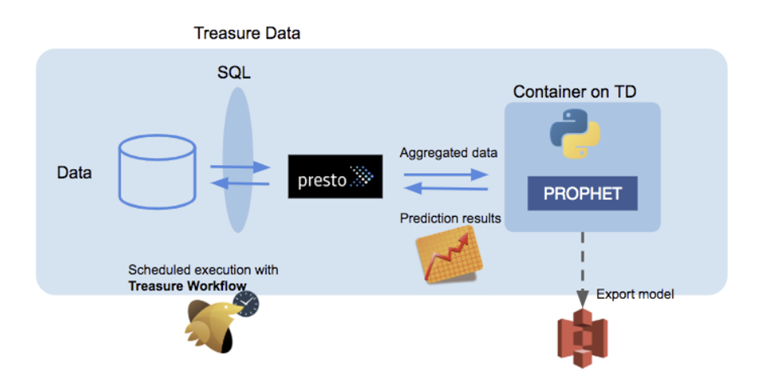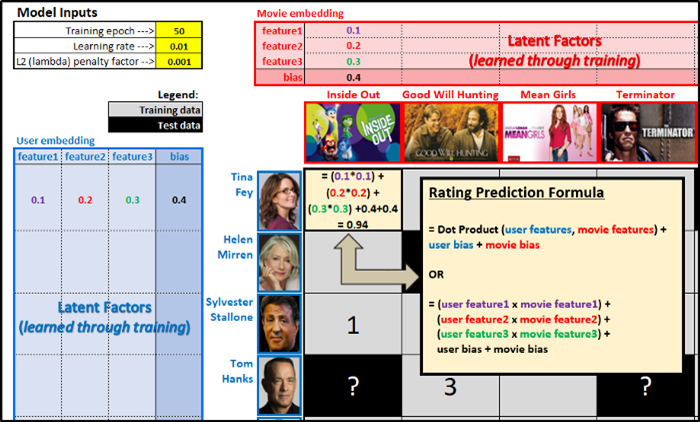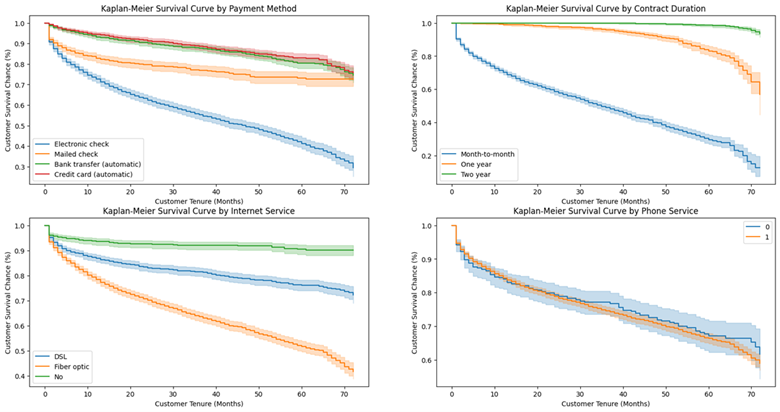# 机器学习聚类算法之K-Means

## K-Means算法

K-Means算法，也被称为K-平均或K-均值算法，是一种广泛使用的聚类算法。K-Means算法是聚焦于相似的无监督的算法，以距离作为数据对象间相似性度量的标准，即数据对象间的距离越小，则它们的相似性越高，则它们越有可能在同一个类簇。之所以被称为K-Means是因为它可以发现k个不同的簇，且每个簇的中心采用簇中所含值的均值计算而成。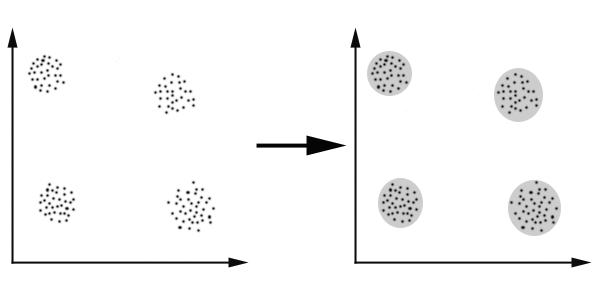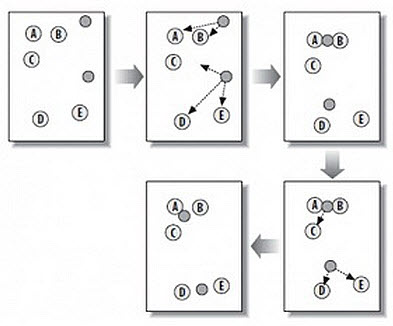• 随机在图中取K（这里K=2）个种子点。
• 然后对图中的所有点求到这K个种子点的距离，假如点Pi离种子点Si最近，那么Pi属于Si点群。（上图中，我们可以看到A,B属于上面的种子点，C,D,E属于下面中部的种子点）
• 接下来，我们要移动种子点到属于他的“点群”的中心。（见图上的第三步）
• 然后重复第2）和第3）步，直到种子点没有移动（我们可以看到图中的第四步上面的种子点聚合了A,B,C，下面的种子点聚合了D，E）。

K-Means算法步骤：

• 初始化常数K，随机初始化k个聚类中心
• 重复计算以下以下过程，知道聚类中心不再改变
• 计算每个样本与每个聚类中心点的距离，将样本划分到最近的中心点
• 计算划分到每个类别中的所有样本特征的均值，并将该均值作为每个类新的聚类中心
• 输出最终的聚类中心以及每个样本所属的类别。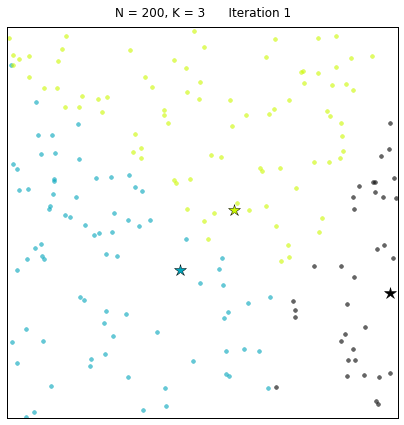Python实现：

import numpy as np
from matplotlib import pyplot as plt

def euclidean_distance(vecA, vecB):
'''计算vecA与vecB之间的欧式距离'''
# return np.sqrt(np.sum(np.square(vecA - vecB)))
return np.linalg.norm(vecA - vecB)

def random_centroids(data, k):
''' 随机创建k个中心点'''
dim = np.shape(data)  # 获取向量的维度
centroids = np.mat(np.zeros((k, dim)))
for j in range(dim):  # 随机生成每一维中最大值和最小值之间的随机数
min_j = np.min(data[:, j])
range_j = np.max(data[:, j]) - min_j
centroids[:, j] = min_j * np.mat(np.ones((k, 1))) + np.random.rand(k, 1) * range_j
return centroids

def KMeans(data, k, distance_func=euclidean_distance):
'''根据k-means算法求解聚类的中心'''
m = np.shape(data)  # 获得行数m
cluster_assment = np.mat(np.zeros((m, 2)))  # 初试化一个矩阵，用来记录簇索引和存储距离平方
centroids = random_centroids(data, k)  # 生成初始化点
cluster_changed = True  # 判断是否需要重新计算聚类中心
while cluster_changed:
cluster_changed = False
for i in range(m):
distance_min = np.inf  # 设置样本与聚类中心之间的最小的距离，初始值为正无穷
index_min = -1  # 所属的类别
for j in range(k):
distance_ji = distance_func(centroids[j, :], data[i, :])
if distance_ji &lt; distance_min:
distance_min = distance_ji
index_min = j
if cluster_assment[i, 0] != index_min:
cluster_changed = True
cluster_assment[i, :] = index_min, distance_min ** 2  # 存储距离平方
for cent in range(k):  # 更新质心，将每个族中的点的均值作为质心
pts_in_cluster = data[np.nonzero(cluster_assment[:, 0].A == cent)]
centroids[cent, :] = np.mean(pts_in_cluster, axis=0)
return centroids, cluster_assment

def show_cluster(data, k, centroids, cluster_assment):
num, dim = data.shape
mark = ['or', 'ob', 'og', 'oy', 'oc', 'om']
for i in range(num):
mark_index = int(cluster_assment[i, 0])
plt.plot(data[i, 0], data[i, 1], mark[mark_index])
for i in range(k):
plt.plot(centroids[i, 0], centroids[i, 1], 'o', markeredgecolor='k', markersize=16)
plt.show()

if __name__ == "__main__":
data = []
f = open("sz.txt", 'r')
for line in f:
data.append([float(line.split(',')), float(line.split(','))])
data = np.array(data)
k = 4
centroids = random_centroids(data, k)
centroids, cluster_assment = KMeans(data, k)
show_cluster(data, k, centroids, cluster_assment)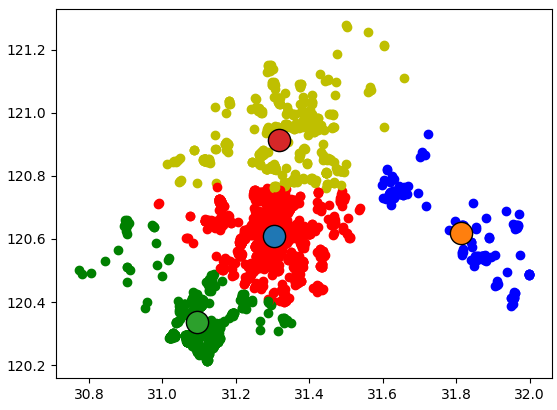## K-Means++算法

k个初始化的质心的位置选择对最后的聚类结果和运行时间都有很大的影响，因此需要选择合适的k个质心。如果仅仅是完全随机的选择，有可能导致算法收敛很慢。K-Means++算法就是对K-Means随机初始化质心的方法的优化。K-Means++算法与K-Means算法最本质的区别是在k个聚类中心的初始化过程。

K-Means++算法的基本思路

K-Means++算法在聚类中心的初始化过程中的基本原则是使得初始的聚类中心之间的相互距离尽可能远，这样可以避免出现上述的问题。K-Means++算法的初始化过程如下所示：

• 在数据集中随机选择一个样本点作为第一个初始化的聚类中心
• 选择出其余的聚类中心：
• 计算样本中的每一个样本点与已经初始化的聚类中心之间的距离，并选择其中最短的距离，记为d_i
• 选择一个新的数据点作为新的聚类中心，选择的原则是：距离较大的点，被选取作为聚类中心的概率较大
• 重复上述过程，直到k个聚类中心都被确定
• 对k个初始化的聚类中心，利用K-Means算法计算最终的聚类中心。

Python实现：

def nearest(data, cluster_centers, distance_func=euclidean_distance):
min_dist = np.inf
m = np.shape(cluster_centers)  # 当前已经初始化的聚类中心的个数
for i in range(m):
d = distance_func(data, cluster_centers[i, ])  # 计算point与每个聚类中心之间的距离
if min_dist &gt; d:  # 选择最短距离
min_dist = d
return min_dist

def get_centroids(data, k, distance_func=euclidean_distance):
m, n = np.shape(data)
cluster_centers = np.mat(np.zeros((k, n)))
index = np.random.randint(0, m)  # 1、随机选择一个样本点为第一个聚类中心
cluster_centers[0, ] = np.copy(data[index, ])
d = [0.0 for _ in range(m)]  # 2、初始化一个距离的序列
for i in range(1, k):
sum_all = 0
for j in range(m):
d[j] = nearest(data[j, ], cluster_centers[0:i, ], distance_func)  # 3、对每一个样本找到最近的聚类中心点
sum_all += d[j]  # 4、将所有的最短距离相加
sum_all *= random()  # 5、取得sum_all之间的随机值
for j, di in enumerate(d):  # 6、获得距离最远的样本点作为聚类中心点
sum_all -= di
if sum_all &gt; 0:
continue
cluster_centers[i] = np.copy(data[j, ])
break
return cluster_centers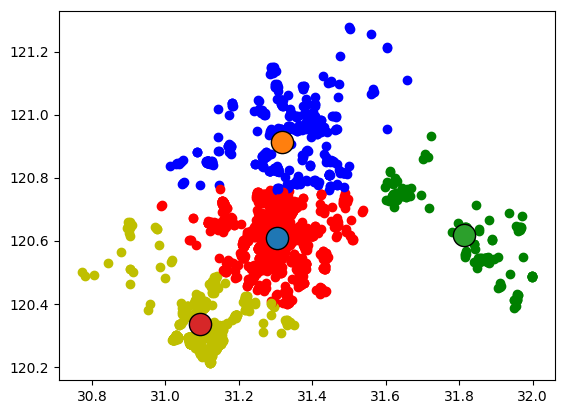## 二分K-Means算法

K-Means是一种最大期望算法，这类算法会在“期望”和“最大化”两个阶段不断迭代。比如K-Means的期望阶段是将各个点分配到它们所“期望”的分类中，然后在最大化阶段重新计算中心点的位置。再继续讨论K-Means算法之前，我想先介绍一下登山式算法。

• 在山上随机选取一个点作为开始
• 向高处爬一点
• 重复第2步，直到没有更高的点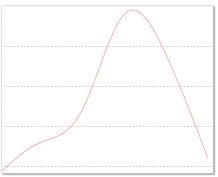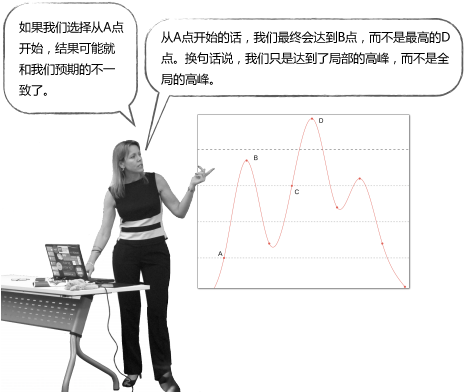K-Means算法收敛，但是聚类效果较差的原因是，K-Means算法收敛到了局部最小值，而非全局最小值（局部最小值指结果还可以但并非最好结果，全局最小值是可能的最好结果）。

$$SSE = \sum_{i=1}^{k}\sum_{x\epsilon c_i}{dist(c_i,x)^2}$$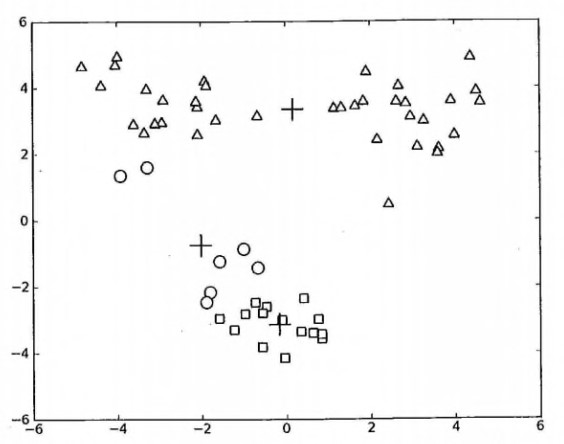• 将所有点看成一个簇
• 当簇数目小于 k 时，对于每一个簇
• 计算总误差
• 在给定的簇上面进行 KMeans 聚类（k=2）
• 计算将该簇一分为二之后的总误差
• 选择使得误差最小的那个簇进行划分操作

Python实现：

def bisecting_KMeans(data, k, distance_func=euclidean_distance):
m = np.shape(data)  # 获得行数m
cluster_assment = np.mat(np.zeros((m, 2)))  # 初试化一个矩阵，用来记录簇索引和存储距离平方
centroid0 = np.mean(data, axis=0).tolist()  # 质心初始化为所有数据点的均值
cent_list = [centroid0]  # 初始化只有1个质心的list
for j in range(m):  # 计算所有数据点到初始质心的距离平方误差
cluster_assment[j, 1] = distance_func(np.mat(centroid0), data[j, :]) ** 2  # 计算距离的评分
while len(cent_list) &lt; k:
lowest_SSE = np.inf
for i in range(len(cent_list)):
pts_in_curr_cluster = data[np.nonzero(cluster_assment[:, 0].A == i), :]  # 获取当前簇i下的所有数据点
centroid_mat, split_cluster_ass = KMeans(pts_in_curr_cluster, 2, distance_func)  # 将当前簇i进行二分kMeans处理
sse_split = sum(split_cluster_ass[:, 1])  # 将二分 kMeans 结果中的平方和的距离进行求和
sse_not_split = sum(
cluster_assment[np.nonzero(cluster_assment[:, 0].A != i), 1])  # 将未参与二分kMeans分配结果中的平方和的距离进行求和
if (sse_split + sse_not_split) &lt; lowest_SSE:  # 总（未拆分和已拆分）误差和越小，越相似，效果越优化，划分的结果更好
best_cent_to_split = i
best_new_cents = centroid_mat
best_cluster_ass = split_cluster_ass.copy()
lowest_SSE = sse_split + sse_not_split
# 找出最好的簇分配结果
best_cluster_ass[np.nonzero(best_cluster_ass[:, 0].A == 1), 0] = len(cent_list)  # 调用二分 kMeans 的结果，默认簇是 0,1. 当然也可以改成其它的数字
best_cluster_ass[np.nonzero(best_cluster_ass[:, 0].A == 0), 0] = best_cent_to_split  # 更新为最佳质心
# 更新质心列表
cent_list[best_cent_to_split] = best_new_cents[0, :].tolist()  #更新原质心list中的第i个质心为使用二分kMeans后bestNewCents的第一个质心
cent_list.append(best_new_cents[1, :].tolist())  # 添加 bestNewCents 的第二个质心
cluster_assment[np.nonzero(cluster_assment[:, 0].A == best_cent_to_split),:] = best_cluster_ass  # 重新分配最好簇下的数据（质心）以及SSE
return np.mat(cent_list), cluster_assment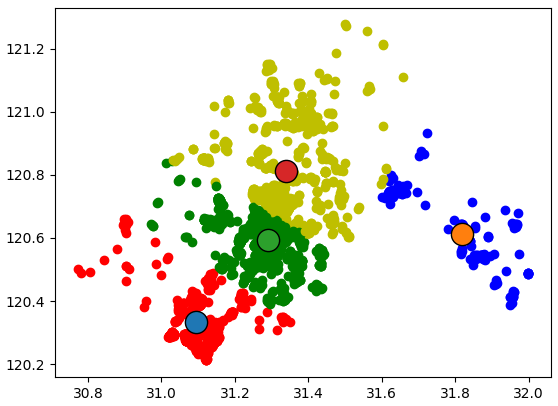## Mini Batch K-Means算法

Mini Batch K-Means算法是K-Means算法的变种，采用小批量的数据子集减小计算时间，同时仍试图优化目标函数，这里所谓的小批量是指每次训练算法时所随机抽取的数据子集，采用这些随机产生的子集进行训练算法，大大减小了计算时间，与其他算法相比，减少了k-均值的收敛时间，小批量k-均值产生的结果，一般只略差于标准算法。

• 从数据集中随机抽取一些数据形成小批量，把他们分配给最近的质心
• 更新质心

Mini Batch K-Means比K-Means有更快的 收敛速度，但同时也降低了聚类的效果，但是在实际项目中却表现得不明显，有差异的基本都是聚类边界上的点。这是一张k-means和mini batch k-means的实际效果对比图。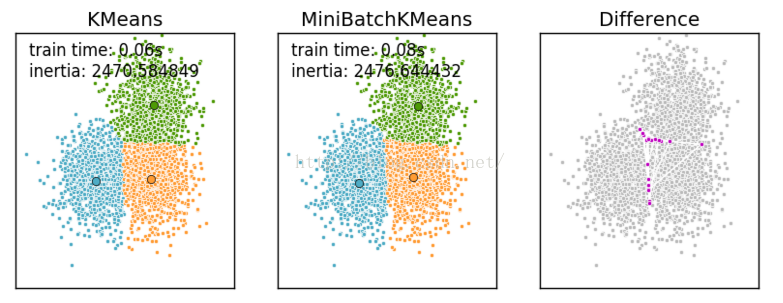## Elkan K-Means算法

Elkan K-Means利用了两边之和大于等于第三边,以及两边之差小于第三边的三角形性质，来减少距离的计算。

• 对于一个样本点x和两个质心$\mu _{j_1}$、$\mu _{j_2}$，如果我们预先计算出这两个质心之间的距离$D(j_1,j_2)$，如果发现$2D(x,j_1) \leq D(j_1,j_2)$，那么我们便能得到$D(x,j_1)\leq D(x,j_2)$。此时我们不再计算$D(x,j_2)$，也就节省了一步距离计算。
• 对于一个样本点x和两个质心$\mu _{j_1}$、$\mu _{j_2}$，我们能够得到$D(x,j_2)\leq max\{0,D(x,j1)-D(j_1,j_2)\}$。这个从三角形的性质也很容易得到。

Elkan K-Means迭代速度比传统K-Means算法迭代速度有较大提高，但如果我们的样本特征是稀疏的，或者有缺失值的话，此种方法便不再使用。

## K-Means小结

K-Means是个简单实用的聚类算法，这里对K-Means的优缺点做一个总结。

K-Means的主要优点有：

• 原理比较简单，实现也是很容易，收敛速度快（在大规模数据集上收敛较慢，可尝试使用Mini Batch K-Means算法）。
• 聚类效果较优。
• 算法的可解释度比较强。
• 主要需要调参的参数仅仅是簇数k。

K-Means的主要缺点有：

• K值的选取不好把握（实际中K值的选定是非常难以估计的，很多时候，事先并不知道给定的数据集应该分成多少个类别才最合适。下篇博客专门讲这个）
• 对于不是凸的数据集比较难收敛（于密度的聚类算法更加适合，比如DBSCAN算法，后面再介绍DBSCAN算法）
• 如果各隐含类别的数据不平衡，比如各隐含类别的数据量严重失衡，或者各隐含类别的方差不同，则聚类效果不佳。
• 采用迭代方法，得到的结果只是局部最优。（可以尝试采用二分K-Means算法）
• 对噪音和异常点比较敏感。（改进1：离群点检测的LOF算法，通过去除离群点后再聚类，可以减少离群点和孤立点对于聚类效果的影响；改进2：改成求点的中位数，这种聚类方式即K-Mediods聚类）
• 对初始值敏感（初始聚类中心的选择，可以尝试采用二分K-Means算法或K-Means++算法）
• 时间复杂度高O(nkt)，其中n是对象总数，k是簇数，t是迭代次数。
• 只适用于数值型数据，只能发现球型类簇。